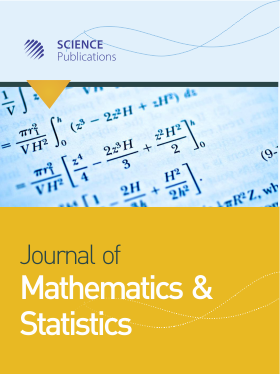Frequency: Continuous
ISSN: 1549-3644 (Print)
ISSN: 1558-6359 (Online)
Editorial Open Access

# Chlodowsky Type (λ, q)-Bernstein Stancu Operator of Korovkin-Type Approximation Theorem of Rough I-Core of Triple Sequences

Ayhan Esi1, Subramanian Nagarajan2 and Kemal Ozdemir3
• 1 Department of Mathematics, Malatya Turgut Ozal University, Turkey
• 2 Department of Mathematics, Sastra University, Turkey
• 3 Department of Mathematics, Inonu University, Turkey

## Editorial

In this study, we obtain a Korovkin-type approximation theorem for Chlodowsky type (λ, q)-Bernstein Stancu operator of rough I-convergent of triple sequences of positive linear operators of two variables from Hw (K) to Cw (K). We introduce and study some basic properties of Korovkin-type approximation theorem for Chlodowsky type (λq)-Bernstein Stancu operator of rough I-convergent of triple sequence spaces and also study the set of all Korovkin-type approximation theorem for Chlodowsky type (λq)− Bernstein Stancu operator of rough I-limits of triple sequence spaces and the relation between analyticness and Korovkin-type approximation theorem for Chlodowsky type (λq)-Bernstein Stancu operator of rough I-core of triple sequence spaces.

Journal of Mathematics and Statistics
Volume 18 No. 1, 2022, 71-77

DOI:

Submitted On: 2 March 2022 Published On: 5 July 2022

How to Cite: Esi, A., Nagarajan, S. & Ozdemir, K. (2022). Chlodowsky Type (λ, q)-Bernstein Stancu Operator of Korovkin-Type Approximation Theorem of Rough I-Core of Triple Sequences. Journal of Mathematics and Statistics, 18(1), 71-77. https://doi.org/10.3844/jmssp.2022.71.77

• 854 Views